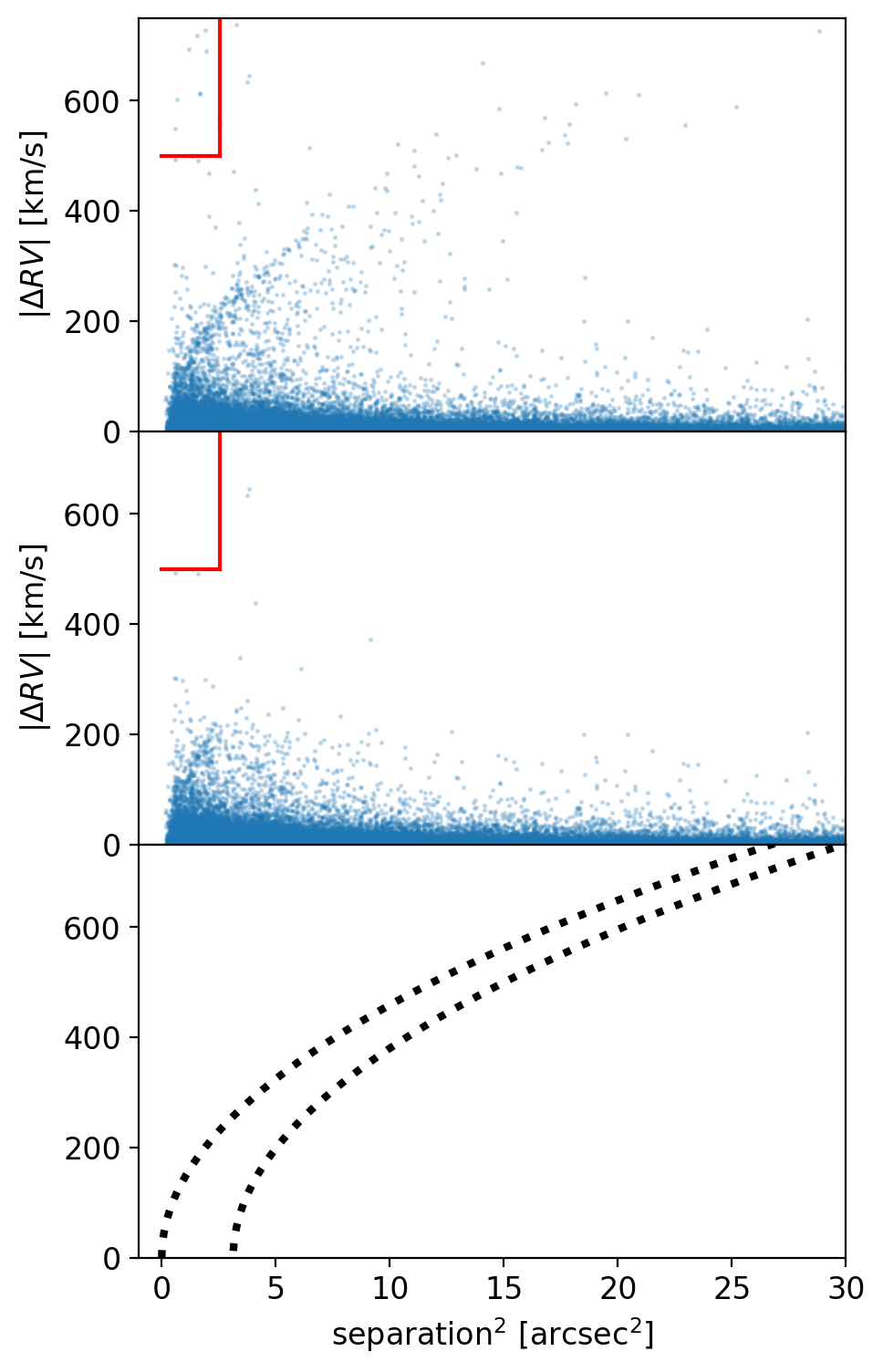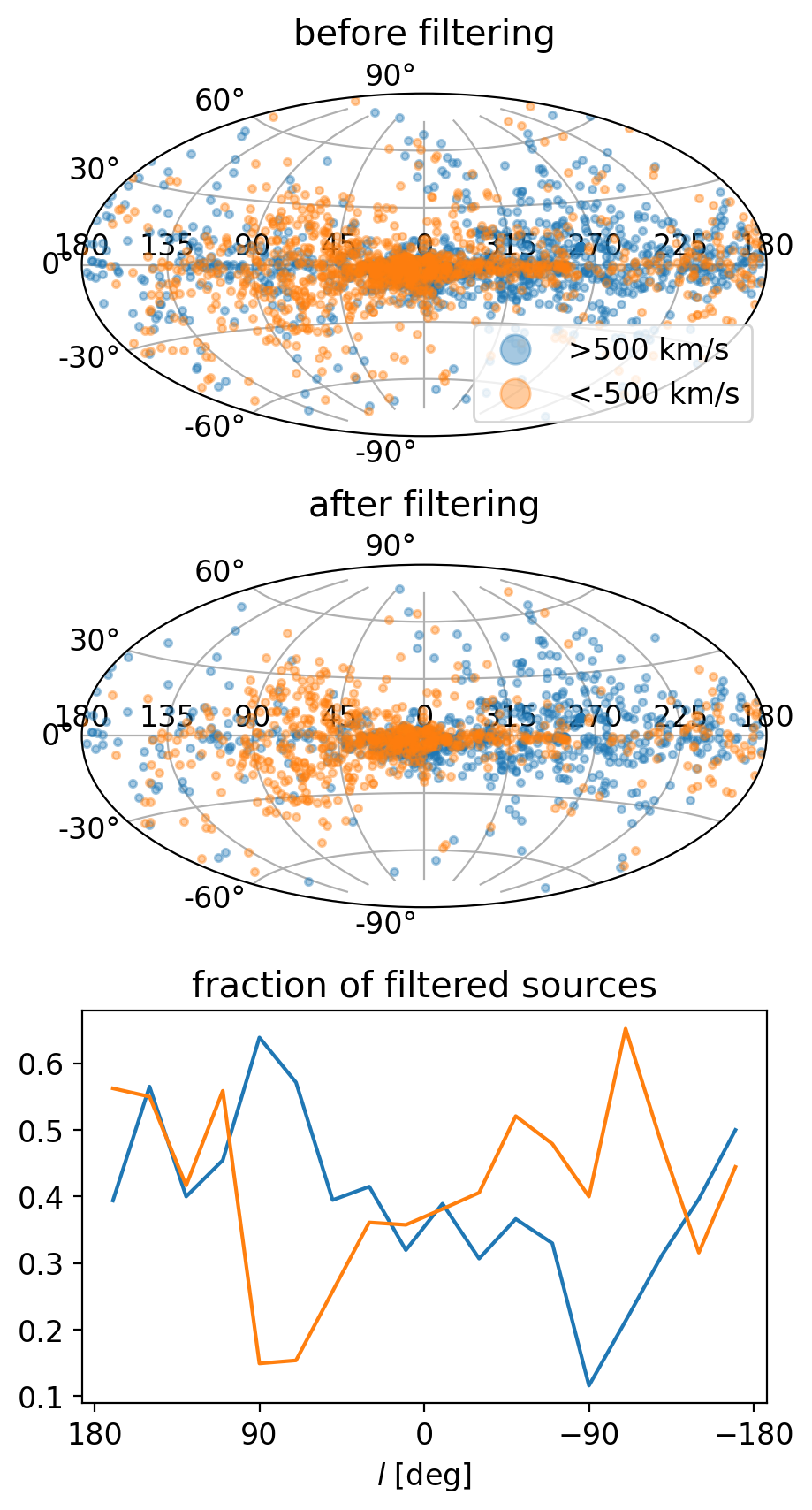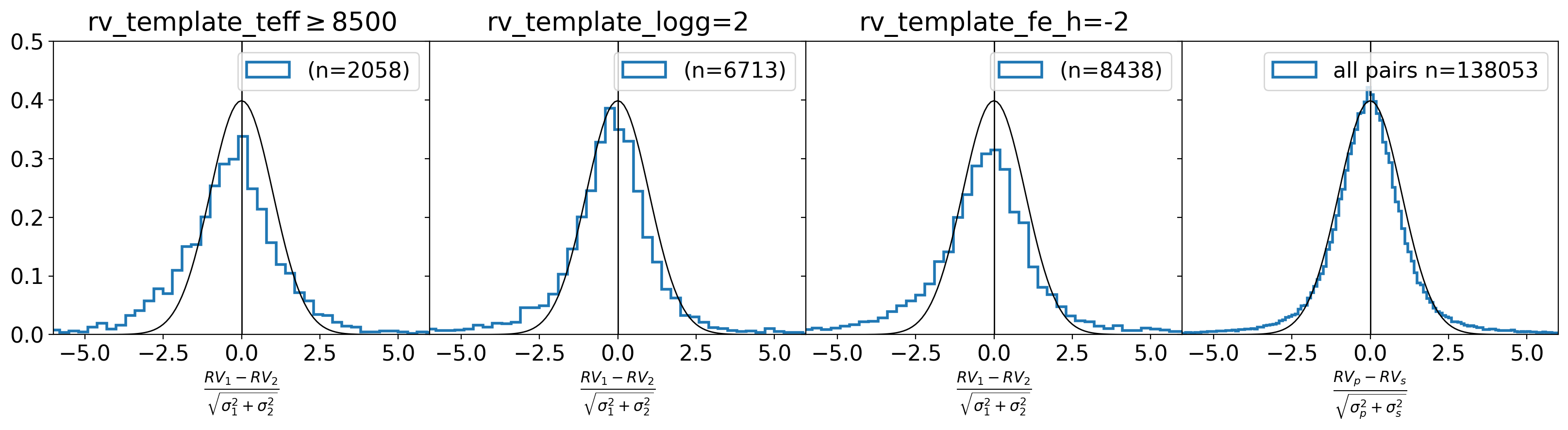### gaia data release 3 documentation

The most relevant tests for the internal consistency for the radial_velocity for Gaia DR3 have been the ones concerning the consistency for binary pairs; the issue of spurious, high radial velocities; and the contamination by closeby sources, also leading to spurious radial velocities.Figure 14.25: Differences in radial velocities of members of binary pairs as a function of the square of the angular separation in arcsec2 (top) and after filtering (middle). The red lines enclose one of the criteria used to filter the problematic cases. In the bottom panel the predicted lines of correlation are shown (see details in Babusiaux et al. 2023). The data shows fronts matching these predictions.

We detected erroneous radial velocities when the lines from a neighbour source are confused with the lines of the fainter target source, with a difference in velocity in proportion to the separation, causing two different dependencies depending on the dominant scanning direction in that sky area. More details are given in Babusiaux et al. (2023) where we examine the velocity difference of nearby sources. Among all the close pairs that suffer from this issue there are also the binary pairs taken from the catalogue by El-Badry et al. (2021), for which the same double dependency of the radial velocity difference with separation (Figure 14.25) is seen. The sources showing these correlations have been only partially eliminated (middle panel).Figure 14.26: Sky distribution of sources with radial velocities |R⁢V|>500⁢km⁢s-1 before (top) and after filtering (middle). Blue and orange sources have positive and negative R⁢V, respectively. The bottom panel shows the fraction of positive (blue) and negative (orange) radial velocities that have been filtered out at each longitude.

As in previous releases, we examined the sources with large radial velocities. Spuriously large radial velocities result from the issue of contamination by nearby sources explained above. In Figure 14.26 we examine the sky distribution of the sources with high radial velocity (above $500$ $\rm\,km\,s^{-1}$). The unfiltered sample is shown in the top panel separated into positive (blue) and negative (orange) radial velocities. We see mostly positive and negative radial velocities for negative galactic longitudes (or 180-360${}^{\circ}$) and positive galactic longitudes (0-180${}^{\circ}$), respectively, resulting from the galactic rotation and the solar motion. We also see higher density of these sources in the bulge region. These patterns appear also in mock catalogues such as GOG (not shown here). The middle panel shows the distribution after filtering and the bottom panel shows the fraction of positive and negative radial velocities that have been filtered out. It is reassuring that most of the eliminated sources are positive high radial velocities that were at positive longitude and negative ones at negative longitudes (thus, mostly removing stars that did not follow the expected trend). There were 2408 sources with these velocities and 1544 remain after the filter (-34%).Figure 14.27: Normalised differences of the radial velocities of members of binary pairs for different templates used. The first three panels are for pairs in which a certain template was used for one of the sources of the pair. The last panel shows the differences for all pairs. The black line shows the expected differences if the errors were Gaussian. The number n is the number of pairs in each panel.

The binary pairs allowed us to do other consistency tests. We computed the differences in radial velocity in the pairs normalised to the uncertainties by the expression:

 $\frac{RV_{p}-RV_{s}}{\sqrt{\sigma^{2}_{p}+\sigma^{2}_{s}}},$ (14.26)

where $p$ and $s$ stand for primary and secondary, respectively. The overall sample shows a small bias of $-0.036$ ($-0.1\rm\,km\,s^{-1}$ without normalising to the errors) and a dispersion of 2.5 (Figure 14.27, right panel). To quantify the number of pairs with normalised differences above $10\sigma$ before and after the filtering, we have changed the denominator of Equation 14.26 to $\sqrt{\sigma^{2}_{p}+\sigma^{2}_{s}+10^{2}}$ to generously account for intrinsic variations in binaries. The number of pairs with normalised differences above $10\sigma$ has diminished considerably (from 956 to 326) after the filtering.

We have also examined these differences for the different templates used (rv_template_teff, rv_template_logg, rv_template_fe_h) to derive the radial velocity (Figure 14.27). In this case the normalised differences are computed with respect to the source having this template (which we name 1), independently whether it is the primary or secondary. The first three panels show the cases where larger biases are seen. In particular, we see negative biases for templates with high effective temperatures, for low surface gravity and low metallicity. In all panels we see wings of larger differences above the expected Gaussian error curve (in black).

Continuing with the templates, in Figure 14.28 we have analysed the differences between the template values and the derived parameters by GSP-Phot (top tow), GSP-Spec (middle row) and ESP-HS (bottom row) for a random subsample of stars with radial velocity of about 150 000 sources. Significant differences are seen in the $\log g$ from GSP-Phot, $T_{\rm eff}$ and $[\rm Fe/H]$ from GSP-Spec and $T_{\rm eff}$ from ESP-HS. Possible biases may be introduced by a wrong choice of template.Figure 14.28: Comparison between template values (horizontal axis) and derived astrophysical parameters (vertical) for different methods: GSP-Phot (top row), GSP-Spec (middle), ESP-HS (bottom row).